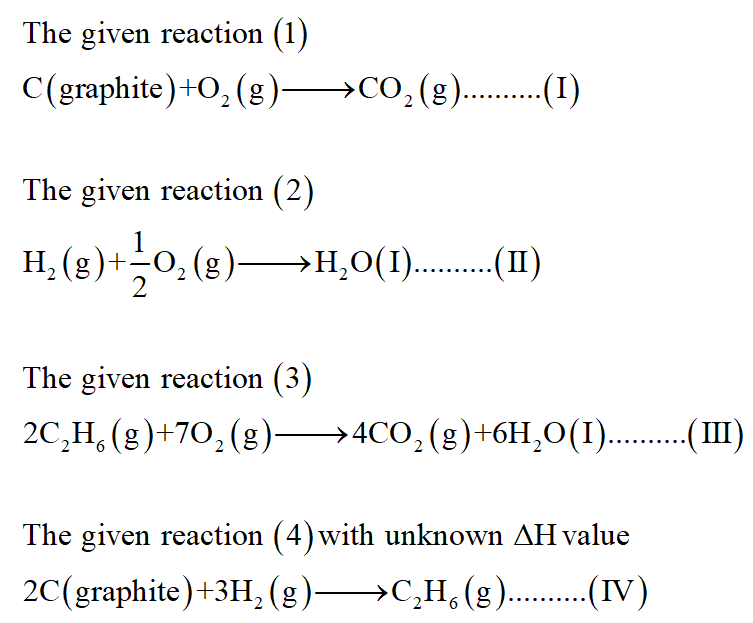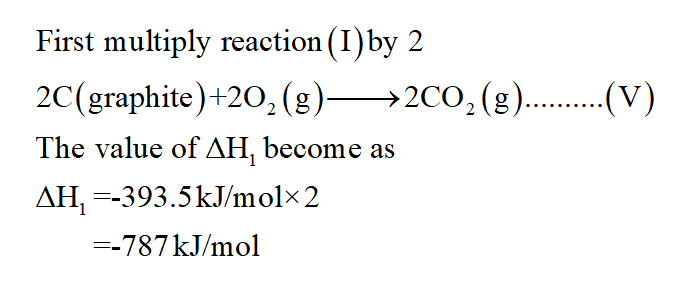# Enter your answer in the provided box. From the following data, C(graphite) + O2(g) → CO2(g) ΔHo rxn = −393.5 kJ/mol H2(g) + 1 2O2(g) → H2O(l) ΔHo rxn = −285.8 kJ/mol 2C2H6(g) + 7O2(g) → 4CO2(g) + 6H2O(l) ΔHo rxn = −3119.6 kJ/mol calculate the enthalpy change for the reaction below: 2 C(graphite) + 3H2(g) → C2H6(g)

Question
2 views

From the following data,

C(graphite) + O2(g

CO2(g)

Δ

H

 o rxn

= −393.5 kJ/mol

H2(g) +

 1 2

O2(g

H2O(l)

Δ

H

 o rxn

= −285.8 kJ/mol

2C2H6(g) + 7O2(g

4CO2(g) + 6H2O(l)

Δ

H

 o rxn

= −3119.6 kJ/mol

calculate the enthalpy change for the reaction below:

2 C(graphite) + 3H2(g

C2H6(g)

check_circle

Step 1

Given:

∆H1= -393.5 kJ/mol

∆H2= -285.8 kJ/mol

∆H3= -3116.6 kJ/mol

Step 2

To determine the enthalpy change for given reaction:Step 3...

### Want to see the full answer?

See Solution

#### Want to see this answer and more?

Solutions are written by subject experts who are available 24/7. Questions are typically answered within 1 hour.*

See Solution
*Response times may vary by subject and question.
Tagged in